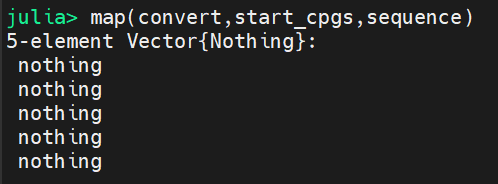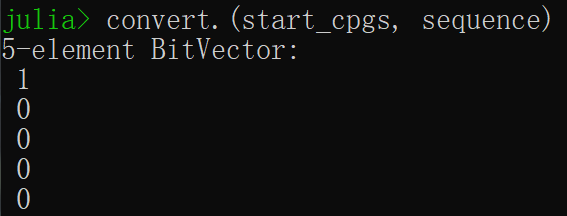# map函数问题

``````function convert(y,string)
if length(string) > y+1
s=string[y+1:y+2]
if s=="CG"
return true
else
return false
end
end
end
``````
``````start_cpgs=[0,3,6,12,22]
sequence="CGTTGGTGTAGGTGTAGAGAGGTGTTTTGTGTTGGTGTAGGTGTAG"
map(convert,start_cpgs,sequence)
```````map` 和向量化对字符串的作用规则不一样。前者把字符串拆成列表，后者当成标量。

``````f(i,j) = (i,j)
map(f, [1, 2, 3], "abc") # (1, a) (2, b) (3, c)
f.([1, 2, 3], "abc") # (1, abc), (2, abc), (3, abc)
``````

convert 这个写法也有点奇怪

• 判断为否时，将返回nothing，返回类型不稳定
• 这个 if else 不如直接写 `return s == 'CG'```````julia> function convert(y,string)
if length(string) > y+1
s=string[y+1:y+2]
return s=="CG" # 这里的值正巧就是true/false
end
end

``````
``````julia> .+([1,2,3,4], 5)
4-element Vector{Int64}:
6
7
8
9

julia> .+([1,2,3,4], [3,4,5,6])
4-element Vector{Int64}:
4
6
8
10
``````